# Complete probability formula

(diff) ← Older revision | Latest revision (diff) | Newer revision → (diff)

A relationship enabling one to calculate the unconditional probability of an event via its conditional probabilities with respect to events forming a complete set of alternatives.

More precisely, let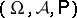be a probability space, and let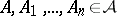be events for which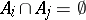for,,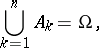andfor all. Then one has the complete probability formula: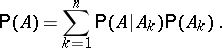The complete probability formula also holds when the number of events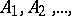is infinite.

The complete probability formula holds for mathematical expectations. Let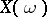,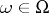, be a random variable on, let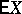be its mathematical expectation and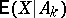the conditional mathematical expectations with respect to events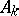which form a complete set of alternatives. ThenA complete set of alternatives is also called a partition of the sample space. A collection of eventsforms a partition if the events are disjoint, have positive probability and if their union is the sample space.